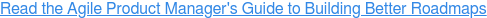# Fibonacci Agile Estimation

## What is Fibonacci Agile Estimation?

Agile estimation refers to a way of quantifying the effort needed to complete a development task. Many agile teams use story points as the unit to score their tasks. The higher the number of points, the more effort the team believes the task will take.

The Fibonacci sequence is one popular scoring scale for estimating agile story points. In this sequence, each number is the sum of the previous two in the series. The Fibonacci sequence goes as follows: 0, 1, 1, 2, 3, 5, 8, 13, 21, 34, 55, 89… and so on.

Fibonacci agile estimation refers to using this sequence as the scoring scale when estimating the effort of agile development tasks.## Why Use the Fibonacci Sequence for Agile Estimation?

Agile consultant Mike Cohn uses a helpful metaphor to explain why the Fibonacci sequence works well for estimating story points.

In his article on Fibonacci agile estimation, Cohn asks us to imagine holding a one-kilogram weight (2.2 pounds) in one hand and a two-kilogram weight (4.4 pounds) in the other. Without looking, could we determine which hand had a more substantial weight? Yes, easily. One is twice as heavy as the other.

But if those two weights were 20kg and 21kg, Cohn explains, we’d have more difficulty knowing which was heavier. In both scenarios, the difference in weight is one kilogram. But as we get into 20kg territory (45 pounds), the difference in the weights will need to be greater so that our brains can perceive it.

This is why Cohn recommends using the Fibonacci sequence for estimating agile story points. The numbers your team can choose from takes larger jumps as the sequence progresses, but they grow at a consistent rate — each number representing about a 60% jump. Cohn points out that even as the numbers get huge, our brains can still perceive the difference if the next number is 60% greater than the previous one.

## How Does Fibonacci Agile Estimation Work in Practice?

Imagine your team wanted to estimate the effort needed to build a new widget in your app. Everyone agreed that this task would rate a high level of difficulty and take a long time to complete it.

But now imagine your team used a linear, even-number scoring scale for story point estimation: 2, 4, 6, 8, 10… up to 50. Even if everyone agreed this new widget would be on the high end of the point scale, could you all agree whether to assign it 42 points? How about 46? Or 48?

As the numbers get higher on this scoring scale, you will find it more difficult to determine the right number because there are too many options, and the numbers at the high end aren’t distinct enough from each other.

Moreover, remember that the goal with these story points is only to estimate the level of effort. There is no reason to try to zero in on the perfect story-point score. These numbers are just a guide to help your team gauge how much time a task will take and how many resources you will need to devote to it. This is why no viable agile estimation scale uses decimals.

If your team was using the Fibonacci sequence to estimate the effort to develop this new widget, you would have only a few numbers to choose from at the top end of the scale: 34, 55, or 89. (This is where your Fibonacci agile scale would stop.)

If you do the math, you’ll see Cohn is correct that each of these numbers jumps about 60% above the previous one in the sequence. And as you can see, it would be much easier to reach a consensus on whether your widget represented a 34-point task, or 55 points, or 89.